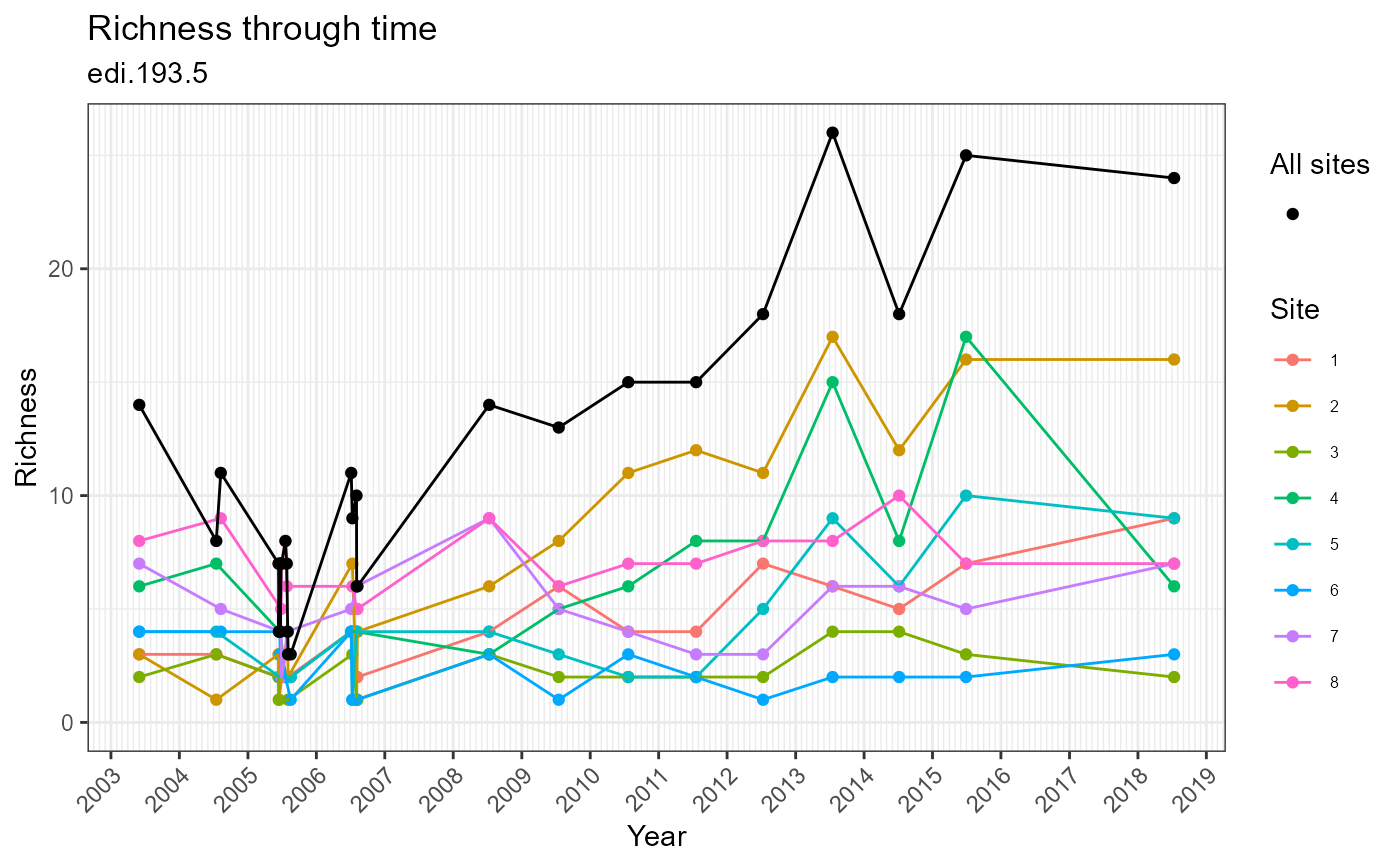Plot diversity (taxa richness) through time

plot_taxa_diversity(
data,
id = NA_character_,
time_window_size = "day",
observation = NULL,
alpha = 1
)

Arguments

data (list or tbl_df, tbl, data.frame) The dataset object returned by read_data(), a named list of tables containing the observation table, or a flat table containing columns of the observation table. (character) Identifier of dataset to be used in plot subtitles. Is automatically assigned when data is a dataset object containing the id field, or is a table containing the package_id column. (character) Define the time window over which to aggregate observations for calculating richness. Can be: "day" or "year" (tbl_df, tbl, data.frame) DEPRECATED: Use data instead. (numeric) Alpha-transparency scale of data points. Useful when many data points overlap. Allowed values are between 0 and 1, where 1 is 100% opaque. Default is 1.

Value

(gg, ggplot) A gg, ggplot object if assigned to a variable, otherwise a plot to your active graphics device

Details

The data parameter accepts a range of input types but ultimately requires the 9 columns of the observation table.

Examples

if (FALSE) { # Read a dataset of interest dataset <- read_data("edi.193.5") # Plot the dataset plot_taxa_diversity(dataset) # Plot the dataset with observations aggregated by year plot_taxa_diversity(dataset, time_window_size = "year") # Flatten the dataset, manipulate, then plot dataset %>% flatten_data() %>% dplyr::filter( lubridate::as_date(datetime) > "2007-01-01") %>% plot_taxa_diversity() # Plot from the observation table directly plot_taxa_diversity(dataset$tables$observation) } # Plot the example dataset plot_taxa_diversity(ants_L1)